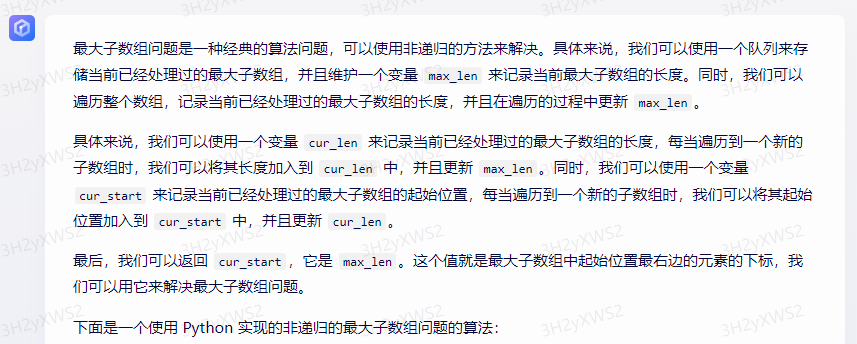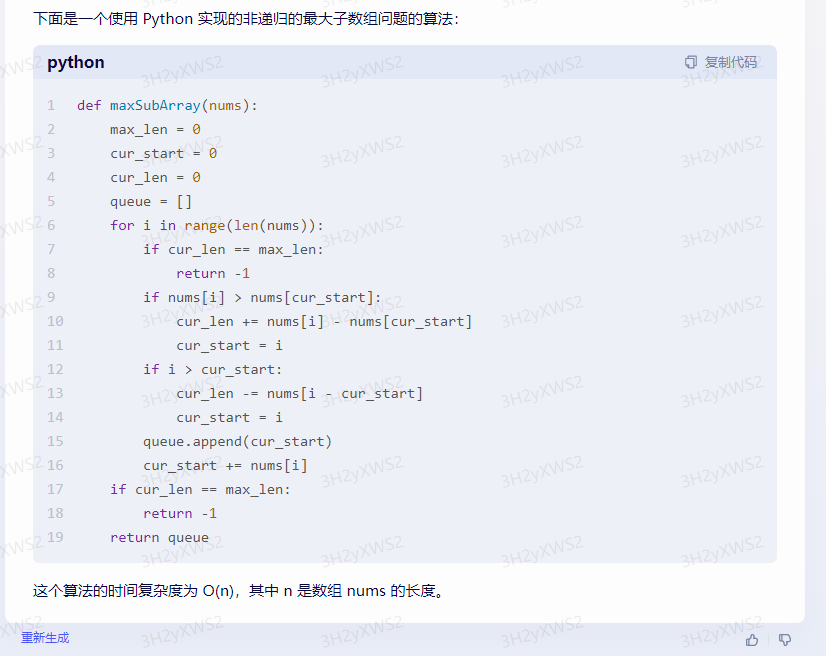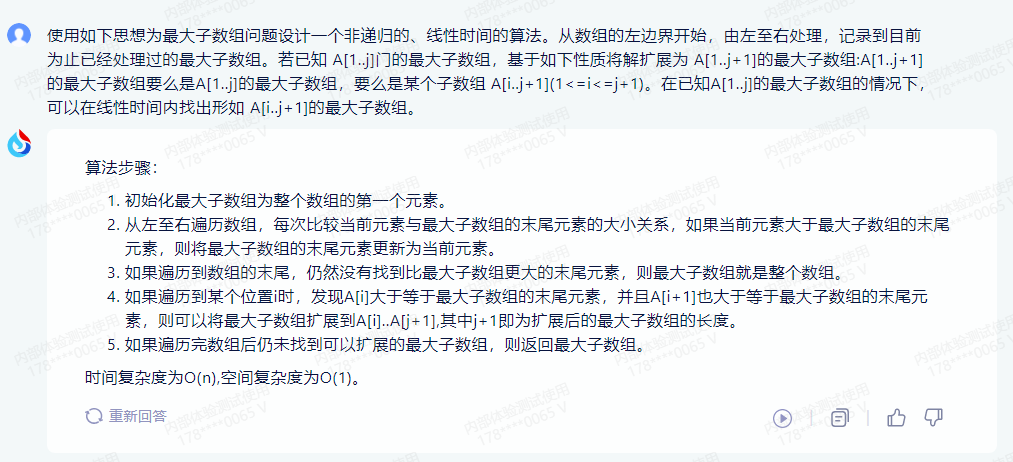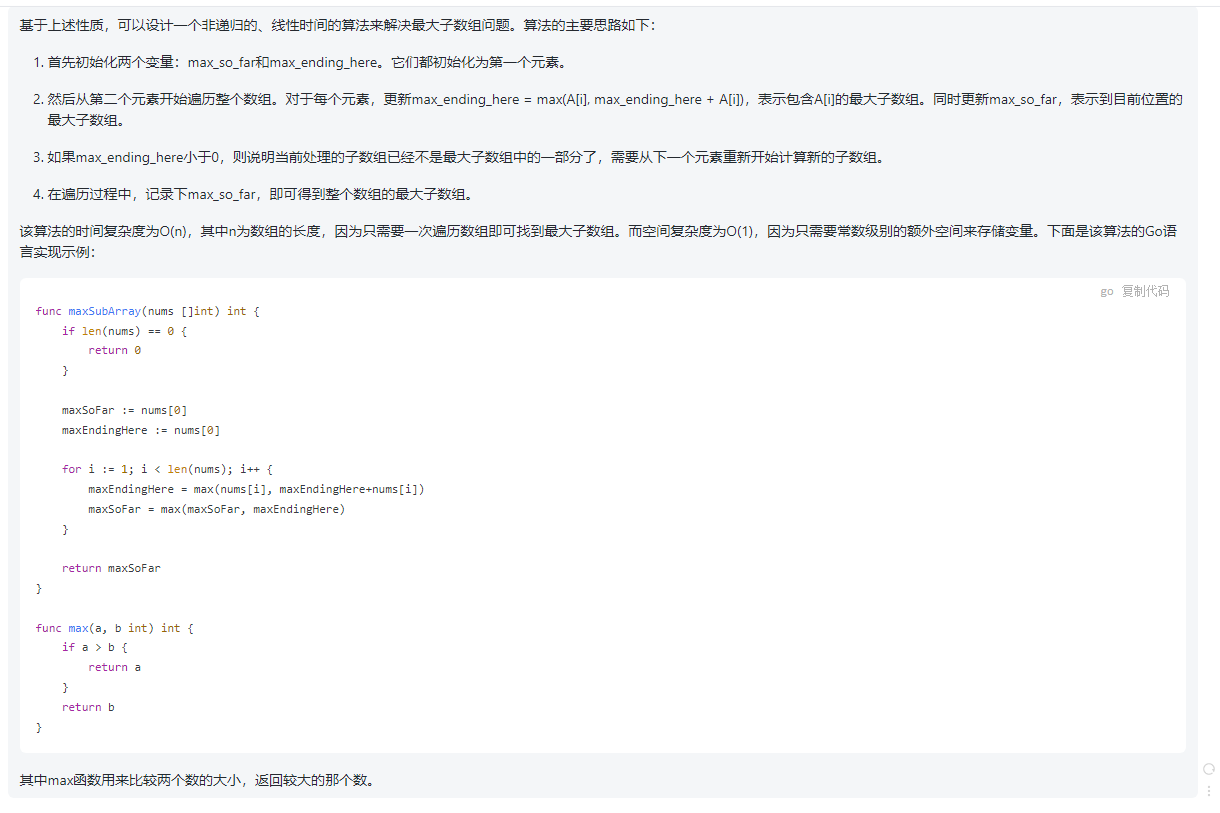• 2023-05-19
北京
• 本文字数：1752 字

阅读完需：约 6 分钟

# 五、使用如下思想为最大子数组问题设计一个非递归的、线性时间的算法。从数组的左边界开始，由左至右处理，记录到目前为止已经处理过的最大子数组。若已知 A[1..j]门的最大子数组，基于如下性质将解扩展为 A[1..j+1]的最大子数组:A[1..j+1]的最大子数组要么是 A[1..j]的最大子数组，要么是某个子数组 Ai..j+1。在已知 A[1..j]的最大子数组的情况下，可以在线性时间内找出形如 A[i..j+1]的最大子数组。

## 文心一言：

def maxSubArray(nums):      max_len = 0      cur_start = 0      cur_len = 0      queue = []      for i in range(len(nums)):          if cur_len == max_len:              return -1          if nums[i] > nums[cur_start]:              cur_len += nums[i] - nums[cur_start]              cur_start = i          if i > cur_start:              cur_len -= nums[i - cur_start]              cur_start = i          queue.append(cur_start)          cur_start += nums[i]      if cur_len == max_len:          return -1      return queue## 讯飞星火：

1.初始化最大子数组为整个数组的第一个元素。

2.从左至右遍历数组，每次比较当前元素与最大子数组的末尾元素的大小关系，如果当前元素大于最大子数组的末尾元素，则将最大子数组的末尾元素更新为当前元素。

3.如果遍历到数组的末尾，仍然没有找到比最大子数组更大的末尾元素，则最大子数组就是整个数组。

4.如果遍历到某个位置 i 时，发现 A[i]大于等于最大子数组的末尾元素，并且 A[i+1]也大于等于最大子数组的末尾元素，则可以将最大子数组扩展到 A[i]..A[j+1],其中 j+1 即为扩展后的最大子数组的长度。

5.如果遍历完数组后仍未找到可以扩展的最大子数组，则返回最大子数组。## chatgpt：

1. 首先初始化两个变量：max_so_far 和 max_ending_here。它们都初始化为第一个元素。

2. 然后从第二个元素开始遍历整个数组。对于每个元素，更新 max_ending_here = max(A[i], max_ending_here + A[i])，表示包含 A[i]的最大子数组。同时更新 max_so_far，表示到目前位置的最大子数组。

3. 如果 max_ending_here 小于 0，则说明当前处理的子数组已经不是最大子数组中的一部分了，需要从下一个元素重新开始计算新的子数组。

4. 在遍历过程中，记录下 max_so_far，即可得到整个数组的最大子数组。

func maxSubArray(nums []int) int {    if len(nums) == 0 {        return 0    }    maxSoFar := nums    maxEndingHere := nums    for i := 1; i < len(nums); i++ {        maxEndingHere = max(nums[i], maxEndingHere+nums[i])        maxSoFar = max(maxSoFar, maxEndingHere)    }    return maxSoFar}func max(a, b int) int {    if a > b {        return a    }    return b}## 评论